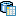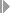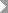Show TOC

# Checking DataUse

A complete, error-free data basis is essential for successful analysis process. This is achieved step by step with the APD. It is then possible to check each individual processing step. The APD allows you to display the data for each step in the analysis process, to calculate intermediate results, and to analyze the quality of the data for some nodes. You can perform this data check already before executing the analysis process.

Features

Displaying Data

Using the Display Data function in the context menu for a node, you can display the data contained in a data source in a table. If an intermediate result has been calculated already, this will be displayed.

Basic Statistics

Using the Display Basic Statistics function in the context menu for a node, you can display the statistics for the selected fields. This information on the data includes histograms, distribution and frequency calculations, simple statistic key figures, such as arithmetical means, standard deviations or correlations.

The way the information about these values is presented depends on the value type of the fields. It differentiates between discrete (DST) and continuous (CNT) fields:

• Discrete means that there are a number of countable values for the field. This applies to almost all characteristics with check table. For characteristics with a large number of values, such as Business Partner, it does not make much sense to have a report for each single value.

Basic statistics for discrete fields: A frequency table of the most frequent values is displayed.

• Continuous means there are is an undefined number of values. A typical example of this is the key figure Revenue.

Basic statistics for continuous fields: A frequency table of the most frequent values is displayed. A value distribution in intervals, the average value, the standard deviation (based on the population) and additional figures are displayed. You can see how these figures are calculated in Formulas for the Calculation of Statistics.

You can select the value type for each selected field. The system does always suggests a logical value type however, continuous for numeric fields and discrete for non-numeric fields.

Example

The Color field, with the values red, blue... receives the value type discrete as the proposal. The Environment field, with values between 0 and 1000, receives the value type continuous as the proposal. If Gender is coded as an integer (1 for male, 2 for female, 0 for sex unknown), you should change the suggested value type from continuous to discrete because calculating the average value does not make sense. If you have chosen continuous for a non-numeric field, the system automatically changes the value type to discrete during execution.

Step Result

Using the Calculate Intermediate Result function in the context menu for a node, you can calculate the data up to this node. The result is saved in a temporary database table and can be helpful for example if you want to try out different options after this node when modeling the analysis process. The intermediate results are also helpful with performance optimization during execution of the analysis process with large amounts of data. If an intermediate result is available for a node, a corresponding iconappears. The intermediate result becomes invalid and is no longer displayed if the node has been changed. This also allows you to delete the intermediate result if it is no longer up to date.

Calculation Summary

Once you have executed the analysis process, you can display additional information about the calculation of the data using the Calculate Calculation Summary function in the context menu for a node. This information can only be called for data mining methods. Depending on the type of transformation, they are comprised of statistical data, probability information or similar. They help to improve evaluation of data quality.

In order to display data and statistics, you need authorization for the simulate activity (48) in authorization object RSANPR.

With the Display Data and Display Basic Statistics functions the complete calculation is performed up to the specified nodes with all data. This can result in short dumps due to exceeding the maximum runtime for dialog processes. In this case, create an intermediate result in the background for the selected node. Start the simulation again once the intermediate result has been calculated.

For larger volumes of data, short dumps can also occur due to memory overflow. If this happends, chooseGotoPerformance Settingsand deselect the Process Data in Memory flag. This flag specifies whether the data is maintained in the main memory during the analysis process or stored temporarily in the database. This flag is set in the standard setting, which means that the data is processed in the main memory. This is the ideal setting when working with small amounts of data. For larger volumes of data, the program can terminate if the data no longer fits in the main memory. If this occurs, deselect this flag. The data is then stored in temporary tables in the database during the analysis process, thus reducing the need for main memory. The generated tables begin with /BIC/000AP.

Tips for Processing Large Volumes of Data:

• Optimize the performance by inserting a filter directly behind the data source.

• Test with mass data: Insert a filter for testing to select a partial quantity of the data. With this restricted volume of data, choose Display Data or Display Basic Statistics. Before executing the analysis process, delete the conditions in the filter.

• If the calculation takes too long, you can end the simulation by choosing Cancel Transaction in the Windows menu in the new window.